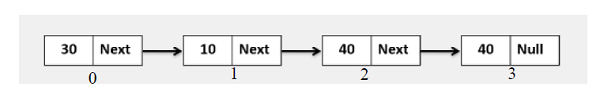# Golang program to insert a node at the ith index node, when the index is at the nth index, i.e., out of bound in the linked list.

Go ProgrammingServer Side ProgrammingProgramming

## Examples## Example

Live Demo

package main
import "fmt"
type Node struct {
value int
next *Node
}
func NewNode(value int, next *Node) *Node{
var n Node
n.value = value
n.next = next
return &n
}
for temp != nil {
fmt.Printf("%d ", temp.value)
temp = temp.next
}
fmt.Println()
}
func InsertNodeAtIthIndex(head *Node, index, data int) *Node{
}
if index == 0{
newNode := NewNode(data, nil)
}
i := 0
preNode := temp
for temp != nil {
if i == index{
newNode := NewNode(data, nil)
preNode.next = newNode
newNode.next = temp
break
}
i++
preNode = temp
temp = temp.next
}
}
func main(){
head := NewNode(30, NewNode(10, NewNode(40, NewNode(40, nil))))
}
Input Linked list is: 30 10 40 40
Inserting new node at 30th index, Linked List is: 30 10 40 40